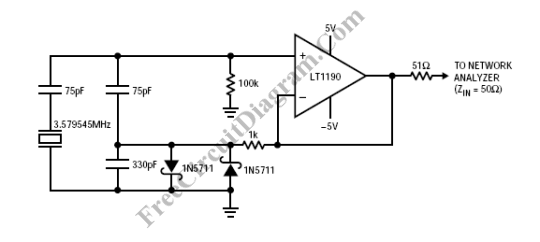# High Frequency Colpitts Crystal OscillatorOp-amps has been widely used in low frequency oscillator (frequency <= 100kHz), but we can actually use it for high frequency crystal oscillator as long as we have an op-amp with the proper bandwidth. An excellent gain stages can be implemented using LT1190 and LT1191 op-amps. Here is the schematic diagram of the circuit:Two Schottky diodes is employed to to limit the gain, so the output will be maintained at approximately +11dBm, which is sufficient to directly drive +7 or +10dBm diode ring mixers. We avoid output stage clipping as the method to maintain this output level since this method introduces distortion and allows the internal nodes to be overdriven. This circuit give a low distortion performance, the  second harmonic is approximately 37dB down, limited primarily by the clipping action of the Schottky diodes. Frequency drift of approximately 0.1ppm/V gives us excellent power supply rejection.  We can use LT1191 to extend the circuit operating range to 20MHz, since the LT1190 gives acceptable performance only up to 10MHz. [Circuit schematic diagram source: Linear Technology Application Notes]